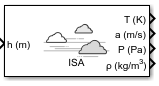# ISA Atmosphere Model

Implement International Standard Atmosphere (ISA)

• Library:
• Aerospace Blockset / Environment / Atmosphere

•## Description

The ISA Atmosphere Model block implements the mathematical representation of the international standard atmosphere values for ambient temperature, pressure, density, and speed of sound for the input geopotential altitude.

The ISA Atmosphere Model and Lapse Rate Model blocks are identical blocks. When configured for ISA Atmosphere Model, the block implements ISA values. When configured for Lapse Rate Model, the block implements lapse rate values.

The ISA Atmosphere Model block icon displays the input and output port labels in metric units.

## Limitations

• Below the geopotential altitude of 0 km and above the geopotential altitude of the tropopause, temperature and pressure values are held.

• Density and speed of sound are calculated using a perfect gas relationship.

## Ports

### Input

expand all

Geopotential height, specified as a scalar or array.

Data Types: `double`

### Output

expand all

Temperature, returned as a scalar or array, in K.

Data Types: `double`

Speed of sound, returned as a scalar or array, in m/s.

Data Types: `double`

Air pressure, returned as a scalar or array, in Pa.

Data Types: `double`

Air density, returned as scalar or array, in kg/m3.

Data Types: `double`

## Parameters

expand all

Customize various atmospheric parameters to be different from the ISA values. Selecting this check box converts the block from ISA Atmosphere Model to Lapse Rate Model.

#### Dependencies

Selecting this check box enables the parameters:

• Acceleration due to gravity (m/s^2)

• Ratio of specific heats

• Characteristic gas constant (J/Kg/K)

• Lapse rate (K/m)

• Height of troposphere (m)

• Height of tropopause (m)

• Air density at mean sea level (Kg/m^3)

• Ambient pressure at mean sea level (N/m^2)

• Lowest altitude (m)

#### Programmatic Use

 Block Parameter: `custom` Type: character vector Values: `'off'` | `'on'` Default: `'off'`

Acceleration from gravity (g). in m/s2, specified as double scalar.

#### Dependencies

This parameter is enabled when the Change atmospheric parameters check box is selected.

#### Programmatic Use

 Block Parameter: `g` Type: character vector Values: double scalar Default: `9.80665`

Ratio of specific heats γ, specified as a double value.

#### Dependencies

This parameter is enabled when the Change atmospheric parameters check box is selected.

#### Programmatic Use

 Block Parameter: `gamma` Type: character vector Values: double scalar Default: `1.4`

Characteristic gas constant (R), specified as double scalar, in J/Kg/K.

#### Dependencies

This parameter is enabled when the Change atmospheric parameters check box is selected.

#### Programmatic Use

 Block Parameter: `R` Type: character vector Values: double scalar Default: `287.0531`

Lapse rate of the troposphere, specified as double scalar, in K/m.

#### Dependencies

This parameter is enabled when the Change atmospheric parameters check box is selected.

#### Programmatic Use

 Block Parameter: `L` Type: character vector Values: double scalar Default: `0.0065`

Height of the troposphere (range of decreasing temperatures), specified as double scalar, in m.

#### Dependencies

This parameter is enabled when the Change atmospheric parameters check box is selected.

#### Programmatic Use

 Block Parameter: `h_trop` Type: character vector Values: double scalar Default: `11000`

Height of the tropopause (range of constant temperature), specified as double scalar, in m.

#### Dependencies

This parameter is enabled when the Change atmospheric parameters check box is selected.

#### Programmatic Use

 Block Parameter: `h_strat` Type: character vector Values: double scalar Default: `20000`

Air density at mean sea level, specified as double scalar, in Kg/m3.

#### Dependencies

This parameter is enabled when the Change atmospheric parameters check box is selected.

#### Programmatic Use

 Block Parameter: `rho0` Type: character vector Values: double scalar Default: `1.225`

Ambient pressure at mean sea level, specified as double scalar, in N/m2.

#### Dependencies

This parameter is enabled when the Change atmospheric parameters check box is selected.

#### Programmatic Use

 Block Parameter: `P0` Type: character vector Values: double scalar Default: `101325`

Ambient temperature at mean sea level (T0), specified as double scalar, in K.

#### Dependencies

This parameter is enabled when the Change atmospheric parameters check box is selected.

#### Programmatic Use

 Block Parameter: `K` Type: character vector Values: double scalar Default: `101325`

Lowest altitude above which temperature and pressure lapse, specified as double scalar, in m. Lowest altitude (m) must be below Height of tropopause.

#### Dependencies

This parameter is enabled when the Change atmospheric parameters check box is selected.

#### Programmatic Use

 Block Parameter: `h0` Type: character vector Values: double scalar Default: `0`

 U.S. Standard Atmosphere., Washington, D.C.: U.S. Government Printing Office, 1976.

## SupportGet trial now